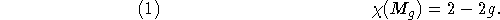Next: Subscripting and Superscripting Up: Commands for Math Mode Previous: Commands for Math Mode

## Numbered Equations

Recall that in-line math is produced by using single dollar signs, $...$, and displayed equations are produced by using double dollar signs, $$...$$. To produce a numbered equation, use the equation environment; for example,

\begin{equation}
\chi(M_g) = 2-2g,
\end{equation}
producesNote that the equation environment puts you in math mode, so there is no need to use $$...$$ around the \begin{equation} ... \end{equation}.

If you want to refer to a numbered equation, you can put a \label{ } command between the \begin{equation} and the \end{equation}. Then the key will be associated with the number of the equation, and you can reference the equation by typing, for example,

...as we see from (\ref{eqnkey}).
See Section 6.8 above for explanations of the \label{ } and \ref{ } commands.

Processed by LaTeX2html